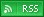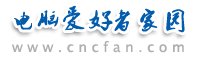电脑爱好者，提供IT资讯信息及各类编程知识文章介绍，欢迎大家来本站学习电脑知识。 最近更新 | 联系我们站内搜索：

# Photoshop快捷键之Photoshop二级快捷键

[文件] ALT+F

+B（批处理）

“[文件] ALT+F”表示“文件”菜单的快键是“ALT+F”；

“自动：ALT+F+U”表示“自动”命令的快捷键是“ALT+F+U”，即它处在“文件”菜单（F）下；

“+B（批处理）”表示“自动”命令（或菜单）下含有多个子命令，其中“批处理”的快捷键是“ATL+F+u+b”，即该命令处于“文件”菜单中的“自动”命令（或菜单）下。

[窗口主菜单]

[子菜单详细操作]

[文件] ALT+F

+B（批处理）；

+P（PDF演试文稿）；

+C（创建快键批处理）。

[编辑] ALT+E

+S（缩放）；

+R（旋转）；

+K（斜切）；

+D（扭曲）；

+P（透视）；

+1（旋转180度）；

+9（顺时针旋转90度）；

+0（逆时针旋转90度）

；+H（水平翻转）；

+（V）垂直翻转。

+U（还原）；

+C（剪贴板）；

+H（历史记录）；

+A（全部）；

[图像]

ALT+I

+B（位图）；

+G（灰度）；

+D（双色调）；

+I（索引颜色）；

+R（RGB颜色）；

+C（CMYK颜色）；

+L（LAB颜色）；

+M（多通道）；

+A（8位通道）；

+N（16位通道）；

+T（颜色表）；

+P（指定配置文件）；

+V（转换为配置文件）；

+c（亮度/对比度）；

+R（匹配颜色）；

+S（可选颜色）；

+X（通道混合器）；

+G（渐变映射）；

+F（照片滤镜 CS功能）；

+W（暗调/高光 CS功能）；

+E（色调均化）；

+T（阈值）；

+P（色调分离）；

+N（变化）

+1（旋转180度）

+9（ 顺时针旋转90度）

+0 （逆时针旋转90度）

[图层]

ALT+L

+B （图层背景）

+A （图层组）

+Y （由链接图层组成的图层组）

+L （图层）

+K （链接图层）

+H （隐藏图层）

+N （混合选项）

+D （投影）

+I （内阴影）

+O （外发光）

+W （内发光）

+B （斜面和浮雕）

+T （光泽）

+V （颜色叠加）

+G （渐变叠加）

+Y （图案叠加）

+K （描边）

+C （拷贝图层样式）

+P （粘贴图层样式）

+S （将图层样式粘贴到链接的图层）

+A （清除图层样式）

+L （全局光）

+R （创建图层）（注：是将图层样式转成图层）

+H （隐藏所有效果）

+F （缩放效果）

+O （纯色）

+G （渐变）

+R （图案）

+L （色阶）

+V （曲线）

+B （色彩平衡）

+C （亮度/对比度）

+H （色相/饱和度）

+S （可选颜色）

+X （通道混合器）

+M （渐变映射）

+I （反相）

+T （阈值）

+P （色调分离）

+C （创建工作路径）

+A （转换为形状）

+H （水平文字方向）

+V （垂直文字方向）

+N （消除锯齿无）

+R （消除锯齿锐化）

+I （消除锯齿明晰）

+S （消除锯齿强）

+M （消除锯齿平滑）

+P （转换为点文本）

+W （文字变形）

+T （文字）

+S （形状）

+F （填充内容）

+V （矢量蒙版）

+L （图层）

+K （链接图层）

+A （所有图层）

+D （扔掉）

+A （应用）

+D （去边）

+B （移去黑色杂边）

+W （移去白色杂边）

[选择]

ALT+S

+B （边界）

+S （平滑）

+E （扩展）

+C （收缩）

[滤镜]ALT+T

=================================================

[视图]

+U （自定）

+C （工作中的CMYK）

+Y （工作中的青版）

+M （工作中的洋红版）

+W （工作中的的黄版）

+B （工作中的的黑版）

+P （工作中的CMY版）

+A （Macintosh RGB）

+I （Windows RGB）

+O （显示器 RGB）

+L （模拟纸白）

+T （模拟墨黑）

+S （标准屏幕模式）

+M （带有菜单的全屏模式）

+F （全屏模式）

（本组命令对应于按连续按下F键，文字工具下除外）

+S （选区边缘）

+L （切片）

+O （注释）

+A （全部）

+N （无）

+I （显示额外选项）

+G （参考线）

+R （网格）

+S （切片）

+D （文档边界）

+A （全部）

+N （无）

[窗口]ALT+W

+D （层叠）

+T （拼贴）

+I （排列图标）

+W （为“未标题-1”新建窗口）

+S （存储工作区）

+D （删除工作区）

+K （复位调板位置）

[使用频率较高的二级快捷键]

[文件][自动][批处理] ALT+F+U+B

[文件][自动][脚本] ALT+F+K

[编辑] ALT+E

+S（缩放）；

+R（旋转）；

+K（斜切）；

+D（扭曲）；

+P（透视）；

+1（旋转180度）；

+9（顺时针旋转90度）；

+0（逆时针旋转90度）

；+H（水平翻转）；

+（V）垂直翻转。

[图像] ALT+I

+G（灰度）；

+D（双色调）；

+I（索引颜色）；

+R（RGB颜色）；

+C（CMYK颜色）；

+c（亮度/对比度）；

+F（照片滤镜 CS功能）；

+W（暗调/高光 CS功能）；

+1 旋转180度

+9 顺时针旋转90度

+0 逆时针旋转90度

[图层]ALT+L

+L 图层

+K 链接图层

+H 隐藏图层

+N 混合选项

+D 投影

+I 内阴影

+O 外发光

+W 内发光

+B 斜面和浮雕

+T 光泽

+V 颜色叠加

+G 渐变叠加

+Y 图案叠加

+K 描边

+C 拷贝图层样式

+P 粘贴图层样式

+S 将图层样式粘贴到链接的图层

+A 清除图层样式

+L 全局光

+R 创建图层（注：是将图层样式转成图层）

+H 隐藏所有效果

+F 缩放效果

+O 纯色

+G 渐变

+R 图案

+L 色阶

+V 曲线

+B 色彩平衡

+C 亮度/对比度

+H 色相/饱和度

+S 可选颜色

+X 通道混合器

+M 渐变映射

+I 反相

+T 阈值

+P 色调分离

+C 创建工作路径

+A 转换为形状

+H 水平（文字方向）

+V 垂直（文字方向）

+N 消除锯齿无

+R 消除锯齿锐化

+I 消除锯齿明晰

+S 消除锯齿强

+M 消除锯齿平滑

+P 转换为点文本

+W 文字变形

+T 文字

+S 形状

+F 填充内容

+V 矢量蒙版

+L 图层

+K 链接图层

+A 所有图层

[选择] ALT+S

+B 边界

+S 平滑

+E 扩展

+C 收缩

[滤镜]ALT+T

[窗口菜单]ALT+W

+K 复位调板位置
 Photoshop教学热门文章排行 网站赞助商